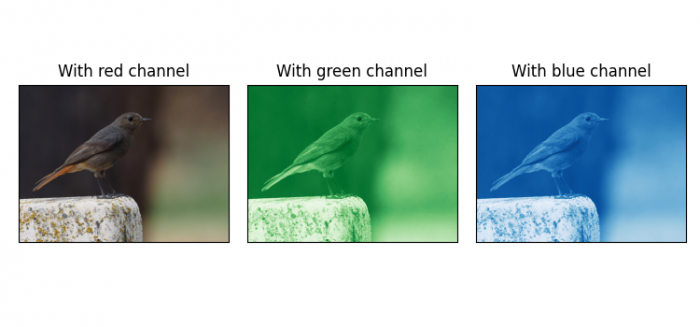# How to show an image in Matplotlib in different colors with different channels?

To slice an image into Red, Green and Blue channels with misc.imread, we can take the following steps −

• Set the figure size and adjust the padding between and around the subplots.
• Read an image from a file into an array.
• Make lists of colormaps and titles.
• Create a figure and a set of subplots.
• Zip the axes, images, titles and colormaps.
• Iterate zipped objs and set the title of each channel image.
• To display the figure, use show() method.

## Example

import matplotlib.pyplot as plt

plt.rcParams["figure.figsize"] = [7.50, 3.50]
plt.rcParams["figure.autolayout"] = True

titles = ['With red channel', 'With green channel', 'With blue channel']
cmaps = [plt.cm.Reds_r, plt.cm.Greens_r, plt.cm.Blues_r]

fig, axes = plt.subplots(1, 3)
objs = zip(axes, (image, *image.transpose(2, 0, 1)), titles, cmaps)

for ax, channel, title, cmap in objs:
ax.imshow(channel, cmap=cmap)
ax.set_title(title)
ax.set_xticks(())
ax.set_yticks(())

plt.show()

## Input Image## Output Image